# Approximate Nearest Neighbors Using Quantizations

This is the third part of 4 parts series on approximate nearest neighbors, but each article is standalone.
Part 1: an introduction to approximate nearest neighbors (ANN).
Part 2: will cover techniques for encoding vectors for ANN.
Part 3: will cover Quantization and Non-Exaustive Search Techniques for ANN.
Part 4: will cover how to choose which algorithms to choose.

# Quantizations Motivation

As we saw in part 1, it’s problematic to compute exact nearest neighbors on high dimensional space due to the fact that it’s not scalable, each query is O(N) and on for most modern applications it’s not practical.

To reduce this complexity of search to in sub-linear time we will be using Approximate Nearest Neighbor algorithms (ANN) using data structure called index, which allows efficient approximate nearest neighbor queries.

Although in modern applications engineers tend to think of linear storage as a “no issue” (due to systems like S3). However in practice, linear storage can become very costly very fast, and the fact that some algorithm requires to load them to RAM and that many systems don’t have separation of storage and compute definitely don’t improve the situation.

This is why Quantization-based algorithms are one of the most common strategies when it comes to ANN.

Quantization is a technique to reduce dataset size (from linear) by defining a function (quantizer) that encode our data into a compact approximated representation.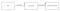Given a dataset construct numeric vectors that represent our dataset, then compress the vectors to an approximated representation

• Quantization Techniques Theory- I will cover the theory behind Quantization, Product Quantization (PQ) and techniques that can be used on top of it, like Inverted File Index Product Quantization (IVPQ). Then I will cover the advantages and disadvantages of these methods.
• Usage- I will show how to use it in practice using the movielens dataset (which contains 100k rows of 64 float).

# Quantization Theory

## Quantization Intuition

The intuition of this method is as follows, we can reduce the size of the dataset by replacing every vector with a leaner approximated representation of the vectors (using quantizer) in the encoding phase.

One way to achieve a leaner approximated representation is to give similar vectors to the same representation. This can be done by clustering similar vectors and represent each of those in the same manner (the centroid representation), the most popular way to so is using k-means.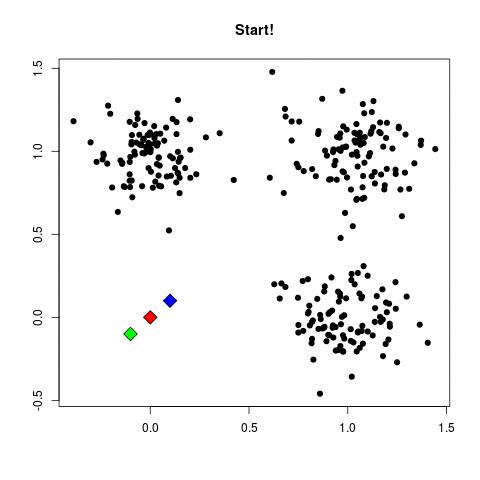An animation demonstrating the inner workings of k-mean, for further information one can go https://towardsdatascience.com/cluster-analysis-create-visualize-and-interpret-customer-segments-474e55d00ebb

Since k-means divide the vectors in space into k clusters, each vector can be represented as one of these k centroids (the most similar one).

This will allow us to represent each vector in a much more efficient way log(k) bit per vector since each vector can be represented in the label of the centroid.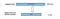In our example, each vector is represented by one of the centroids. since we have 2042 centroids we can represent each vector with 11 bits, as opposed to 4096 ( 1024*32 ).

But, this amazing compaction comes with a great cost, we lost accuracy as we now cant separate the original vector from the centroid.

## Product Quantization Intuition

We saw that using a quantizer like k-means comes with a price, in order to increase the accuracy of our vectors we need to increase drastically the number of centroids, which makes the Quantization phase infeasible in practice.

This what gave birth to Product Quantization, we can increase drastically the number of centroids by dividing each vector into many vectors and run our quantizer on all of these and thus improves accuracy.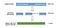In our example, each vector is represented by 8 subvectors which can be represented by one of the centroids. since we have 256 centroids we can represent each matrix in 1 byte, making vector representation 8byte only as oppose to 4096 ( 1024*32 ).

Although it increases the size of the vector a bit compared to the regular quantizer, it’s still O(log(k)) and allows us to increase the accuracy drastically and still work in practice.

Unfortunately in terms of search, even though we can calculate the distances in more efficiently using table look-ups and some addition. We are still going to do an exhaustive search.

The search is done using the following algorithm:

• Construct a table with the calculated distance between each sub-vector and each of the centroids for that sub-vector.
• Calculating approximate distance values for each of the vectors in the dataset, we just use those centroid id’s to look up the partial distances in the table, and sum those up!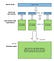In our example, this means that this means building a table of subvector distances with 256 rows (one for each centroid) and 8 columns (one for each subvector). Remember that each database vector is now just a sequence of 8 centroid ids.

## Inverted File Index Intuition

The intuition of the algorithm is, that we can avoid the exhaustive search if we partition our dataset in such a way that on search, we only query relevant partitions (also called Voronoi cells). The reason this tends to work well in practice is since many datasets are actually multi-modal.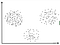We can see that the data here is multi-modal, we can split the data into three partitions without a critical hit to accuracy. Source: https://www.geeksforgeeks.org/ml-classification-vs-clustering/

However, dividing the dataset up this way reduce accuracy yet again, because if a query vector falls on the outskirts of the closest cluster, then it’s nearest neighbors are likely sitting in multiple nearby clusters.

The solution to this issue is simply to search for multiple partitions (this also called probe), searching multiple nearby partitions obviously take more time but it gives us better accuracy.

So as we saw by now it's all about tradeoffs, the number of partitions and the number of the partitions to be searched can be tuned to find the time/accuracy tradeoff sweet spot.

Its important to note thate Inverted File Index is a technique that can be use with other encoding strategies apart from quantization.

## Encoding Residuals Intuition

The intuition of the algorithm is, we want the same number of centroids to give has a more accurate representation. This can be achieved if the vectors are less distinct than they were.

In order, to do so, for each database vector, instead of using the PQ to encode the original database vector we instead encode the vector’s offset from its partition centroid.

However, replace vectors with their offsets will increase search time yet again, as we will need to calculate a separate distance table for each partition we probe since the query vector is different for each partition.

Apparently the trade-off is worth it, though, because the IVFPQ works pretty well in practice.

## Let Put It All Together

The algorithm goes as follow, we partition our dataset ahead of time with k-means clustering to produce a large number of dataset partitions (Inverted Index). Then, for each of the partitions, we run regular product quantization.

Then, for each of the partitions, we are going to break each its’ vector to D/M sub-vectors, this will transform our N x D matrix to D/M matrices of N x M.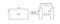In our example, we are going to break each 1024 vector into 8 vectors of 128, thus we look at our dataset as 8 matrices of size 10k x 128.

Then we are going to run the k-means algorithm on each sub-matrix, such that each sub-vector (row) will be connected to one of the k centroids.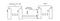In our example, we are going to run k-means on our 8 matrices where k=256. This means, that each of our rows is connected to one of these 256 on each matrix (each row in our original matrix is connected to 8 centroids).

We are going to replace each subvector with the id of the closest matching centroid. This is what we have waited for since we have repeated elements after the previous part, we can now represent each of them with a very small label and keep the actual value only once.

## Pros & Cons

In this section, I will explain the pro & cons of quantization techniques in general.

## Pros

• The only method with sublinear space, great compression ratio (log(k) bits per vector.
• Quantization methods aremore accurate than various hashing methods empirically.
• Support GPUs.

## Cons

• The exact nearest neighbor might be across the boundary to one of the neighboring cells.

It’s, important to note that in part 6 I will cover how to choose which algorithms and get into the maturity of the libraries used and whether they support modern hardware to improve performance.

# Usage

I am going to show how to use faiss library, to do “Approximate Nearest Neighbors Using Product Quantization”. Faiss support many indexing techniques including PQ and IVPQ for the sake of brevity I will use IVPQ.

I am going to use an enriched version of the movielens dataset, which already consists of movie labels and their semantic representation. The entire code can be found as a Jupyter Notebook here.

First, we going to load our dataset which already consists of movie labels and their semantic representation.

`import pickleimport faissdef load_data():    with open('movies.pickle', 'rb') as f:        data = pickle.load(f)    return datadata = load_data()data`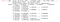As we can see data is actually a dictionary, the name column consists of the movies’ names, and the vector column consists of the movies vector representation.

We are going to create the index class, as you can see most of the logic is in the build method (index creation), where you can control:

• subvector_size — the target size of the subvectors (product quantization phase).
• number_of_partitions — the numbers of partitions to divide the dataset by (Inverted File Index phase).
• search_in_x_partitions — the numbers of partitions to search on (Inverted File Index phase).
`class IVPQIndex():    def __init__(self, vectors, labels):        self.dimension = vectors.shape        self.vectors = vectors.astype('float32')        self.labels = labels    def build(self,               number_of_partition=8,               search_in_x_partitions=2,               subvector_size=8):        quantizer = faiss.IndexFlatL2(self.dimension)        self.index = faiss.IndexIVFPQ(quantizer,                                       self.dimension,                                       number_of_partition,                                       search_in_x_partitions,                                       subvector_size)        self.index.train(self.vectors)        self.index.add(self.vectors)            def query(self, vectors, k=10):        distances, indices = self.index.search(vectors, k)         # I expect only query on one vector thus the slice        return [self.labels[i] for i in indices]`

After I define the IVPQIndex class I can build the index with my dataset using the following snippets.

`index = IVPQIndex(data["vector"], data["name"])index.build()`

Now it’s pretty easy to search, let’s say I want to search for the movies that are most similar to “Nightmare Before Christmas” (its located in the 90 indexes).

`movie_index = 90movie_vector = data['vector'][movie_index:movie_index+1]movie_name = data['name'][movie_index]index.query(movie_vector)`

And that’s it, we have search efficiently using IVPQ for movies similar to “Nightmare Before Christmas” and we got approximated results. It's important to note that faiss support batch queries to increase throughput.

# Last Words

We started this article explaining the theory behind “Approximate Nearest Neighbor” using quantization since this is such a huge topic I “ditched” some techniques and focused and the most common ones (For more one can watch this video).

Then I listed the cons and pros of these algorithms, which is the most important thing to grasp, this will affect whether these can solve your ANN use case. Lastly, I showed how one can actually use these techniques in practice.

I hope I was able to share my enthusiasm for this fascinating topic and that you find it useful, and as always I am open to any kind of constructive feedback.

## More From Medium

### Feature Extraction Techniques: PCA, LDA and t-SNE

#### 12

Welcome to a place where words matter. On Medium, smart voices and original ideas take center stage - with no ads in sight. Watch
Follow all the topics you care about, and we’ll deliver the best stories for you to your homepage and inbox. Explore
Get unlimited access to the best stories on Medium — and support writers while you’re at it. Just \$5/month. Upgrade